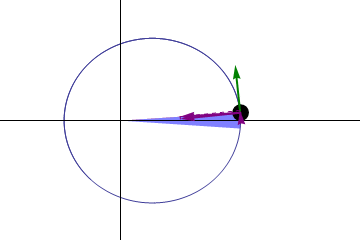# 3.1: Orbital Mechanics

As we discussed in the introduction, orbital mechanics is a branch of planetary physics that uses observations and theories to examine the Earth's elliptical orbit, its tilt, and how it spins. Humans have been studying orbital mechanics since 1543, when Copernicus discovered that planets, including the Earth, orbit the sun, and that planets with a larger orbital radius around their star have a longer period and thus a slower velocity. While these may seem straightforward to us today, at the time these were radical ideas. Johannes Kepler elaborated on Copernicus' ideas in the early 1600's, stating that orbits follow elliptical paths, and that orbits sweep out equal area in equal time, as seen in the animation. The same (blue) area is swept out in a fixed time period. The green arrow is velocity. The purple arrow directed towards the Sun is the acceleration. The other two purple arrows are acceleration components parallel and perpendicular to the velocity.Figure $$\PageIndex{1}$$: Kepler's Second Law for a Planetary Orbit (CC BY-SA 3.0; Gonfer, via Wikimedia)

He also discovered the relationship $$T^2\propto r^3$$, know as Kepler's Third Law, where T is the orbital period of a planet and r is its radius. From this, we can derive a relationship of

$\frac{T_1^2}{T_2^2}=\frac{R_1^3}{R_2^3}$Figure $$\PageIndex{2}$$: Kepler's Third Law

Let's look at how we might determine the orbital period of the satellite. We are given that the orbital period of the moon is 27.3217 days and the radius of the moon is 60+Re where Re is the Earth's radius.

$(\frac{T_s}{T_m})^2=(\frac{R_s}{R_m})^3$

$T_s^2=T_m^2(\frac{R_s}{R_m})^3$

$T_s=T_m(\frac{R_s}{R_m})^{\frac{3}{2}}$

If Rs=20 Re and Ts is given, you can solve this equation. You could also start with Ts and determine the radius. A note about units: you should use what units make sense as long as they are consistent, ie they are the same for both T and R so they cancel out.

We can use the same equation based approach for planets orbiting the sun, moons orbiting planets, and satellites orbiting planets or the sun.

Hohmann Transfer OrbitsFigure $$\PageIndex{3}$$: Hohmann Transfer Orbit

Using the above figure, let's calculate how long it would take to reach mars in the most efficient orbit. We know that the path is an elliptical orbit around the sun, and it grazes mar's orbit at aphelion.Figure $$\PageIndex{4}$$: Perihelion and Aphelion

(Mars is closest to the sun in its orbit at perihelion and farthest at aphelion).

For Hohmann Transfer orbit, the semi-major axis is Rn

$R_n=\frac{1}{2}(R_e+R_m)$

$R_n=\frac{1}{2}(1+1.524)$

$=1.262 AU$

$(\frac{R_n}{R_e})^3=(\frac{T_n}{T_e})^2$

Re= 1 AU and Te=1 earth-year

$(R_n)^3=(T_n)^2$

$(1.262)^3=(T_n)^2$

$2.0099=(T_n)^2$

$T_n=1.412\;years$

This is the full orbit time. A transfer takes only a half orbit.

$\frac{T_n}{2}=0.7088\;years\approx8\;months$

We can use this approach to estimate lots of orbits (R or T) such as: the time to reach the sun (64 days and R=1 AU), the orbit of synchronous satellites (T=1 day), and how far out does Halley's comet go (T=75 years).

Now, let's start connecting what we know about orbits to mass.

In the late 1600s, Newton laid the groundwork for this idea, with his three laws of motion and the law of universal gravitation. As you were likely told in elementary school, legend states that while attempting to escape an outbreak of the bubonic plague, Newton retreated to the countryside, sat in an orchard, and was hit on the head with an apple. This lead him to develop his ideas on gravity, and equate that when an apple falls or planets orbit, the same physics apply. We can derive this mathematically, but we must account for the planet's orbit around the center of gravity, not the sun, (the sun also moves, but not as much).

Newton's Law of Gravity

$$G=6.67x10^{-11}\frac{m^3}{kgs^2}$$

$F=\frac{GMm}{r^2}$Figure $$\PageIndex{5}$$: Centripetal Force

Centripetal force is F=ma or $$F=\frac{mv^2}{r}$$

Now set the forces equal

$\frac{GMm}{r^2}=\frac{mv^2}{r}$

$v^2=\frac{GM}{2}$

What is $$v=\frac{d}{t}=\frac{2\pi r}{T}$$? 2$$\pi$$r is the circumference and T is the orbital period.

Substitute

$(\frac{2\pi r}{T})^2=\frac{GM}{r}$

$\frac{4\pi^2r^2}{T^2}=\frac{GM}{r}$

Solve for T2

$T^2=\frac{4\pi^2}{GM}r^3$

$\frac{T^2}{r^3}=\frac{4\pi^2}{GM}$

Now that we have this equation, let's solve a problem.

Earth Orbiting Sun

M=Msun=1.9891x1030 kg

$$G=6.67x10^{-11}\frac{m^3}{kgs^2}$$

$T^2=Kr^3$

K is the constant of proportionality

$K=\frac{4\pi^2}{GM}$

$=2.97x10^{-19}\frac{s^2}{m^3}$

If r is in AU⇒1 AU=1.49x1011m

T is in earth-years, 1 yr=3.15x107s

K=0.9916$$\approx$$1

This shows that Kepler's Law $$T^2\propto r^3$$ holds true.

But, relating this to the gravitational force gives us something else.

$\frac{4\pi^2r^2}{T^2}=\frac{GM}{r}$

Where $$\frac{GM}{r}$$ is the mass of the object being orbited.

$m=\frac{4\pi^2}{G}\frac{r^3}{T^2}$

In solving real world problems, we can observe the orbital radius and period of the orbiting object. We can then calculate the mass of the object being orbited.

This leads us to calculate the mass of stars, planets with moons, or the mass of a moon with an artificial satellite.

Mass of the Earth

What is the mass of the Earth, Me?

$M_e=\frac{4\pi^2}{G}(\frac{R_{moon}^3}{T_{moon}^2})$

Rmoon=384x106 m

Tmoon=27.3 days=2358720 sec

$M_e=6.02x10^{24}kg$

(actual Me is 5.98x1024kg)